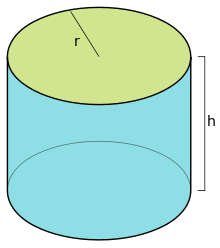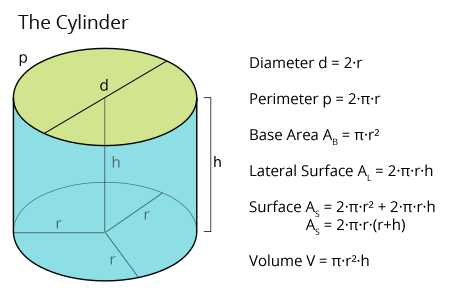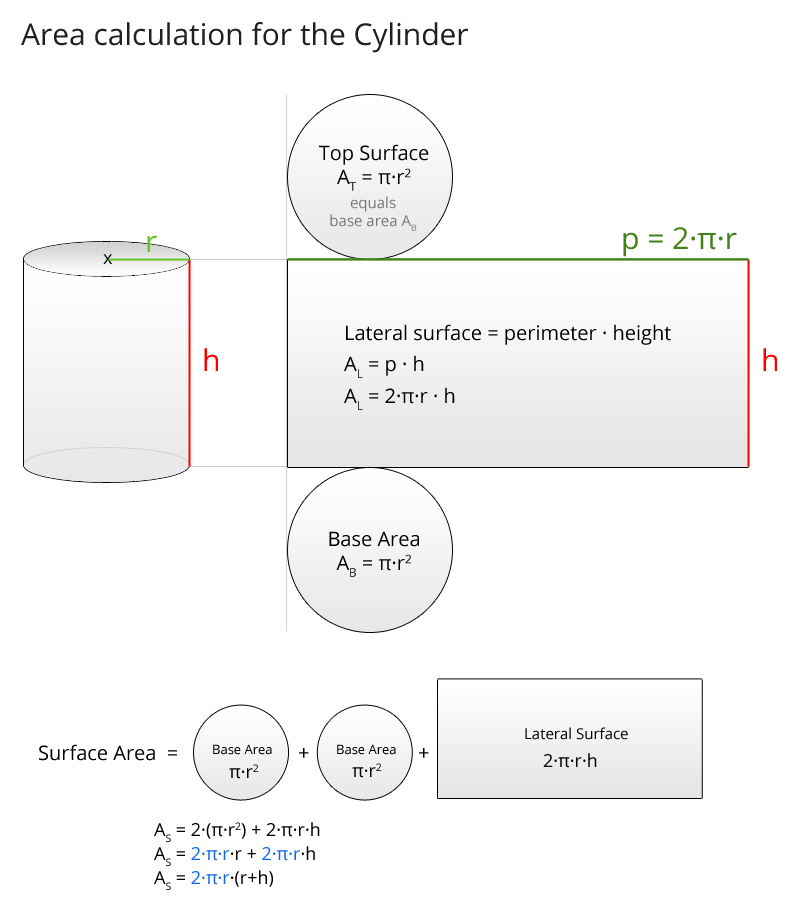# Cylinder Calculator 3D

Cylinder graphic:Results:

Insert two values for the cylinder:

Use keys and for value changes

r h d = 2·r p = 2·π·r AB = π·r2 AL = 2·π·r·h AS = 2·π·r·(r+h) V = π·r2·h

Precision with 3 decimal places

## Interactive 3D-Cylinder

+
Fullscreen

For a better experience we recommend the use of Firefox or Chrome.

### All formulas for the Cylinder at a glance

Here you see the necessary formulas for the calculation of a right circular cylinder:Explanations:

Diameter = 2·radius → d = 2·r

Perimeter = 2·Pi·radius → p = 2·π·r

Base Area = Pi·radius² → AB = π·r²

Lateral Surface = perimeter · height → AL = 2·π·r·h

Surface Area = 2·base area + lateral surface → AS = 2·π·r² + 2·π·r·h = 2·π·r·(r+h)

Volume = base area · height → V = π·r²·h

### What is a cylinder?

Definition: A cylinder (circular cylinder) consists of two parallel opposite circular areas and one rectangular lateral surface, that is perpendicular to the circular areas (base area and top surface). A cylinder is a geometrical shape. Circles are of fundamental importance for this shape, therefore we need the formulas for the circle (circular area and perimeter of a circle).
For the circular cylinder there are the following formulas: The perimeter p is 2·Pi·r (this is the formula for the perimeter of the circle), the base area AB is Pi·r² (this is the formula for the area of a circle), the lateral surface is perimeter times height, AL = p·h and therefore AL = 2·Pi·r·h, the surface area consists of the circular areas on top and on bottom (2 times the base area) and the lateral surface, so surface is AS = 2·AB + AL and thus AS = 2·(π·r²) + (2·π·r·h), while the 2·π·r is often factored out and we get: AS = 2·π·r·(r+h). The volume of the cylinder is base area times height, thus V = AB·h = π·r²·h. cylinder with radius, base area and diameter of the base area. Characteristics of a cylinder.

Example from daily life (form of a cylinder):
Drinking glass, pipe, building stone, engine cylinder, chair leg, pen, form of a pill etc.

#### Flächenberechnung beim Zylinder (Grafik):Possible combinations and calculations for given cylinder values
computable
Height
computable
Solution formula for height
Radius Base area yes no Radius provided Height not computable - Details
Radius Surface area yes yes Radius provided h = AS / 2·π·r – r
See equivalent transformation
See equivalent transformation
See equivalent transformation
Height Perimeter yes yes r = p / 2·π
See equivalent transformation
Height provided
Height Base area yes yes r = AB/π
See equivalent transformation
Height provided
Height Surface area yes yes r1,2 = – h/2 ± √( h2/4 + AS/2·π )
See equivalent transformation
Height provided
Height Lateral surface yes yes r = AL/2·π·h
See equivalent transformation
Height provided
Height Volume yes yes r = ± √( V/h·π )
See equivalent transformation
Height provided
Perimeter Base area yes no r = p / 2·π
See equivalent transformation
Height not computable - Details
Perimeter Surface area yes yes r = p / 2·π
See equivalent transformation
h = AS / p – r
See equivalent transformation
Perimeter Lateral surface yes yes r = p / 2·π
See equivalent transformation
h = AL / p
See equivalent transformation
Perimeter Volume yes yes r = p / 2·π
See equivalent transformation
h = 2·V / u·r
See equivalent transformation
Base area Surface area yes yes r = AB/π
See equivalent transformation
h = r · AS / 2·AB – r
See equivalent transformation
Base area Lateral surface yes yes r = AB/π
See equivalent transformation
h = r · AL / 2·AB
See equivalent transformation
Base area Volume yes yes r = AB/π
See equivalent transformation
h = AL / AB
See equivalent transformation
Surface area Lateral surface yes yes If we have the height, we can calculate
the radius from the formule of the lateral surface:

r = AL / 2·π·h
See equivalent transformation
h = ± √( AL2 / 2·π·AS – 2·π·M )
See equivalent transformation
Surface area Volume yes yes 0 = r3 + AS / -2·π · r + V / π
3 Solutions for the cubic equation
See equivalent transformation
If we have the height, we can calculate
the radius from the formule of the lateral surface:

h = AS / 2·π·r – r
See equivalent transformation
Lateral surface Volume yes yes r = 2·V/AL
See equivalent transformation
If we have the height, we can calculate
the radius from the formule of the lateral surface:

h = AL/2·π·r
See equivalent transformation